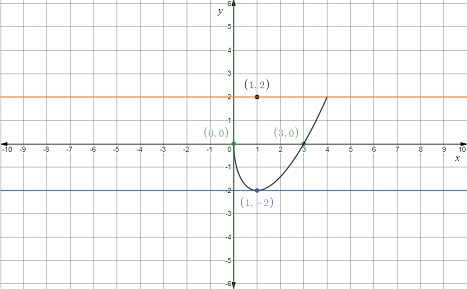# Explain that the given curve has two tangent lines at the given point.### Single Variable Calculus: Concepts...

4th Edition
James Stewart
Publisher: Cengage Learning
ISBN: 9781337687805### Single Variable Calculus: Concepts...

4th Edition
James Stewart
Publisher: Cengage Learning
ISBN: 9781337687805

#### Solutions

Chapter 3.4, Problem 85E

a.

To determine

## Explain that the given curve has two tangent lines at the given point.

Expert Solution

The equation of tangent lines are y=3x+33 and y=3x33 .

### Explanation of Solution

Given:

The given equations are x=t2 and y=t33t . The given point is (3,0) .

Calculation:

Find the slope.

Apply formula dydx=dydtdxdt .

dydt=ddt(t33t)

Apply differencerule (fg)'=f'g' .

dydt=ddt(t3)ddt(3t)

Use derivative rule ddx(xn)=nxn1 .

dydt=3t23dydt=3(t21)

dxdt=ddt(t2)

Use derivative rule ddx(xn)=nxn1 .

dxdt=2t

dydx(slope)=3(t21)2t

Find the value of the parameter t at the point (3,0) .

At x=3

t2=3t=±3

At y=0

t33t=0t(t23)=0t=0andt23=0t2=3t=±3

Now,

t=±3 , because it satisfies both the equations.

Slope at t=3 .

dydx(slope)=3(t21)2t=3[(3)21]2(3)=32(3)=33=33×33=3

Slope at t=3 .

dydx(slope)=3(t21)2t=3[(3)21]2(3)=32(3)=33=33×33=3

There are two different tangents at the given point, because there is two different slope values.

Now use point-slope form for the tangent equations.

yy1=m(xx1)y1=0,x1=3,m=3y0=3(x3)y=3x+33andy1=0,x1=3,m=3y0=3(x3)y=3x33

Hence the equation of tangent lines are y=3x+33 and y=3x33 .

b.

To determine

### Find the horizontal and vertical tangent line points on the curve.

Expert Solution

The tangents are horizontal at the points (2,2) and (2,2) . The tangent is vertical at the point (0,0) .

### Explanation of Solution

Given:

The given equations are x=t2 and y=t33t . The given point is (3,0) .

Calculation:

Find the slope.

Apply formula dydx=dydtdxdt .

dydt=ddt(t33t)

Apply difference rule (fg)'=f'g' .

dydt=ddt(t3)ddt(3t)

Use derivative rule ddx(xn)=nxn1 .

dydt=3t23dydt=3(t21)

dxdt=ddt(t2)

Use derivative rule ddx(xn)=nxn1 .

dxdt=2t

dydx(slope)=3(t21)2t

For the horizontal tangent line.

dydx=03(t21)2t=03(t21)=0t21=0t2=1t=±1t=±1

Substitute t=1 in the given equations.

x=t2=(1)2=1y=t33t=(1)33(1)=2(x,y)=(1,2)

Substitute t=1 in the given equations.

x=t2=(1)2=1y=t33t=(1)33(1)=2(x,y)=(1,2)

For vertical tangent line dxdt=0 .

dxdt=02t=0t=0

Substitute t=0 in the given equations.

x=t2=02=0y=t33t=(0)33(0)=0(x,y)=(0,0)

Hence the tangents are horizontal at the points (2,2) and (2,2) . The tangent is vertical at the point (0,0) .

c.

To determine

Expert Solution

### Explanation of Solution

Given:

The given equations are x=t2 and y=t33t . The given point is (3,0) .

Calculation:

Find the slope.

Apply formula dydx=dydtdxdt .

dydt=ddt(t33t)

Apply difference rule (fg)'=f'g' .

dydt=ddt(t3)ddt(3t)

Use derivative rule ddx(xn)=nxn1 .

dydt=3t23dydt=3(t21)

dxdt=ddt(t2)

Use derivative rule ddx(xn)=nxn1 .

dxdt=2t

dydx(slope)=3(t21)2t

For the horizontal tangent line.

dydx=03(t21)2t=03(t21)=0t21=0t2=1t=±1t=±1

Substitute t=1 in the given equations.

x=t2=(1)2=1y=t33t=(1)33(1)=2(x,y)=(1,2)

Substitute t=1 in the given equations.

x=t2=(1)2=1y=t33t=(1)33(1)=2(x,y)=(1,2)

For vertical tangent line dxdt=0 .

dxdt=02t=0t=0

Substitute t=0 in the given equations.

x=t2=02=0y=t33t=(0)33(0)=0(x,y)=(0,0)

Draw a table for the curve C .

 t x=t2 y=t3−3t 01234 014916 0−221852

Hence the graph of the curve is given below.### Have a homework question?

Subscribe to bartleby learn! Ask subject matter experts 30 homework questions each month. Plus, you’ll have access to millions of step-by-step textbook answers!